# RD Sharma Solutions For Class 7 Maths Exercise 6.1 Chapter 6 Exponents

Get free PDF of RD Sharma Solutions for Class 7 Maths Exercise 6.1 of Chapter 6 Exponents from the given links. Students who aim to top the examinations they can download the PDFs available here. BYJU’S expert team has solved the questions in such a way that learners can understand easily and comfortably. Students who aim to score high in the Maths of Class 7 are advised to practice all the questions present in the RD Sharma Solutions for Class 7 as many times as possible. This exercise includes definition, the meaning of exponents along with how to read exponents. Here students will thorough about how to express a given number in terms of exponents and why it is introduced and so on.

## Download the PDF of RD Sharma Solutions For Class 7 Maths Chapter 6 – Exponents Exercise 6.1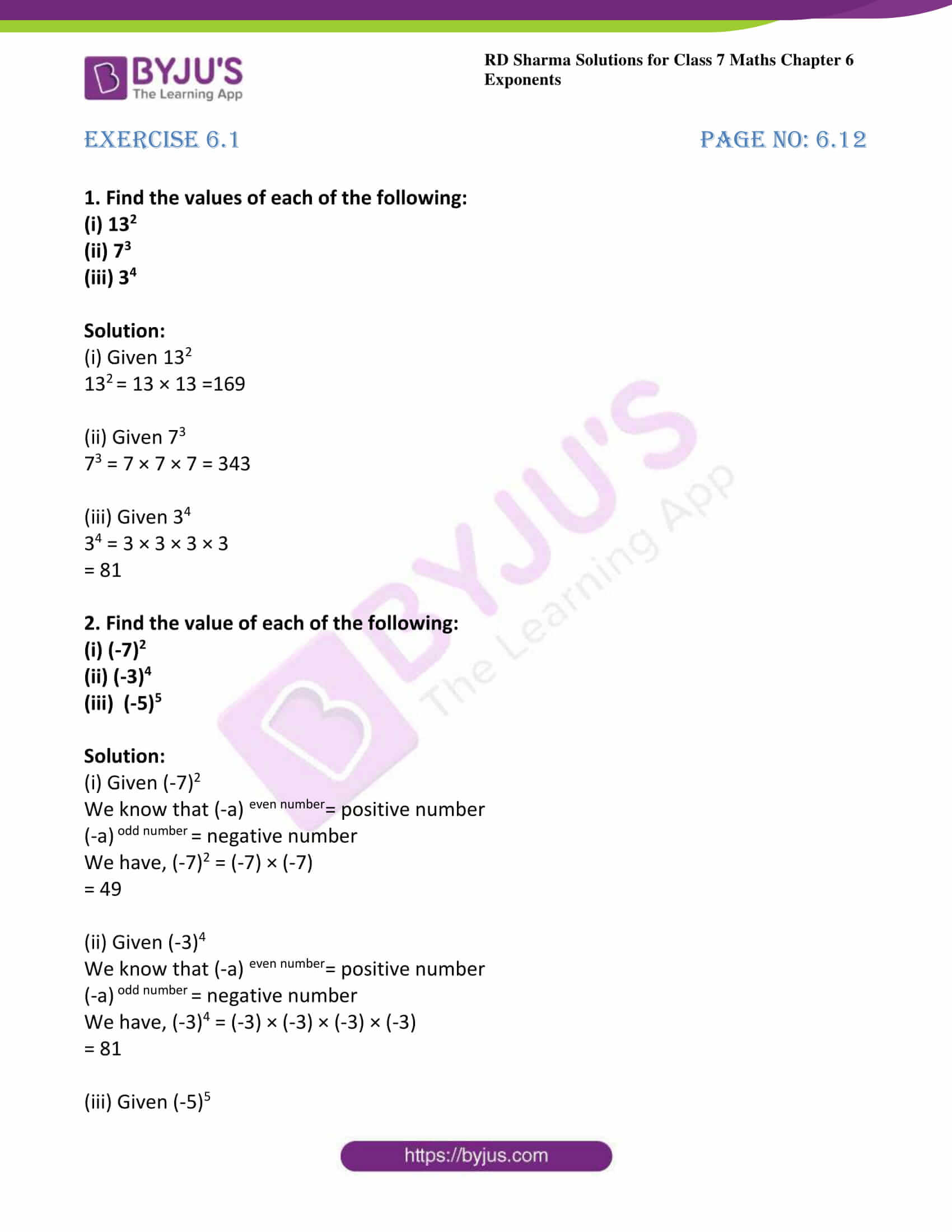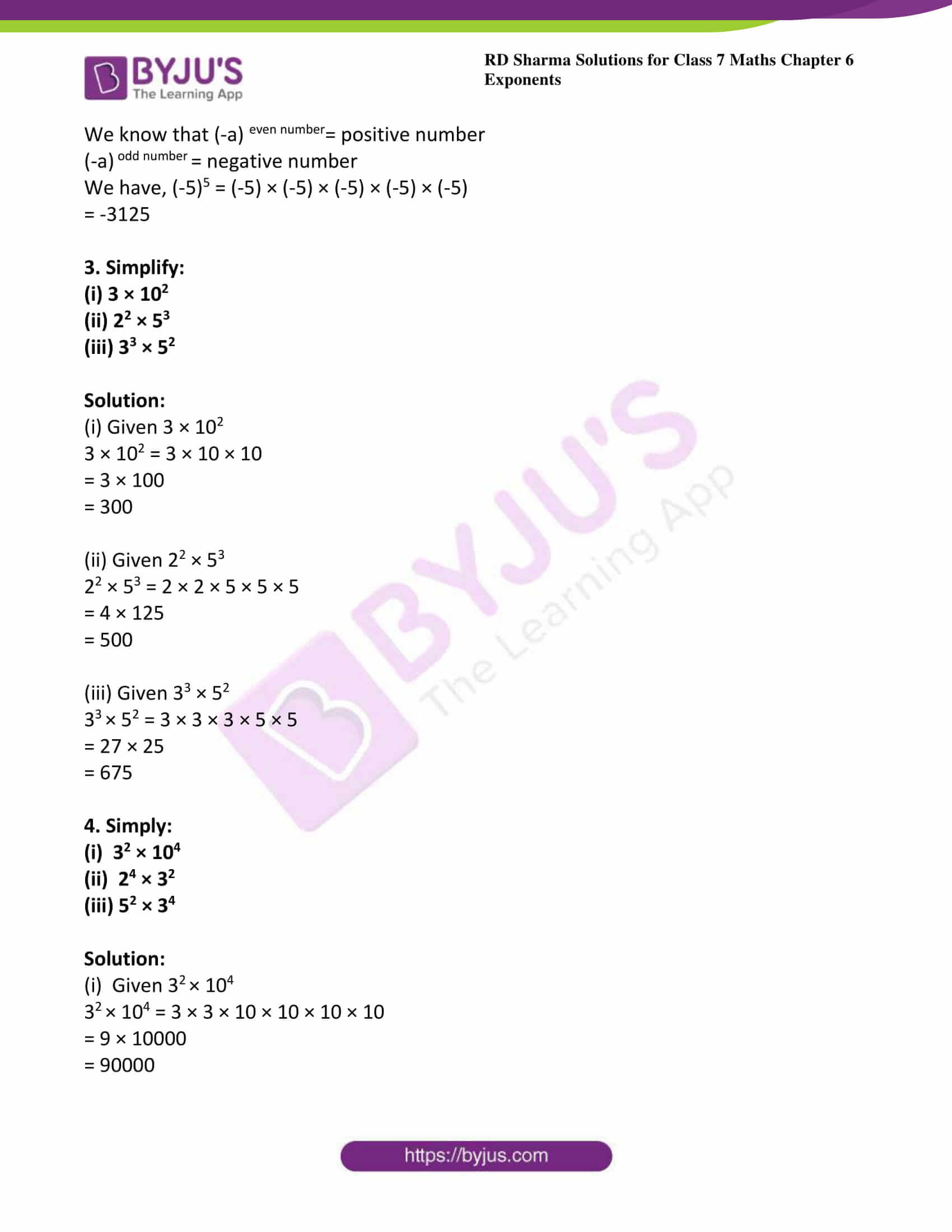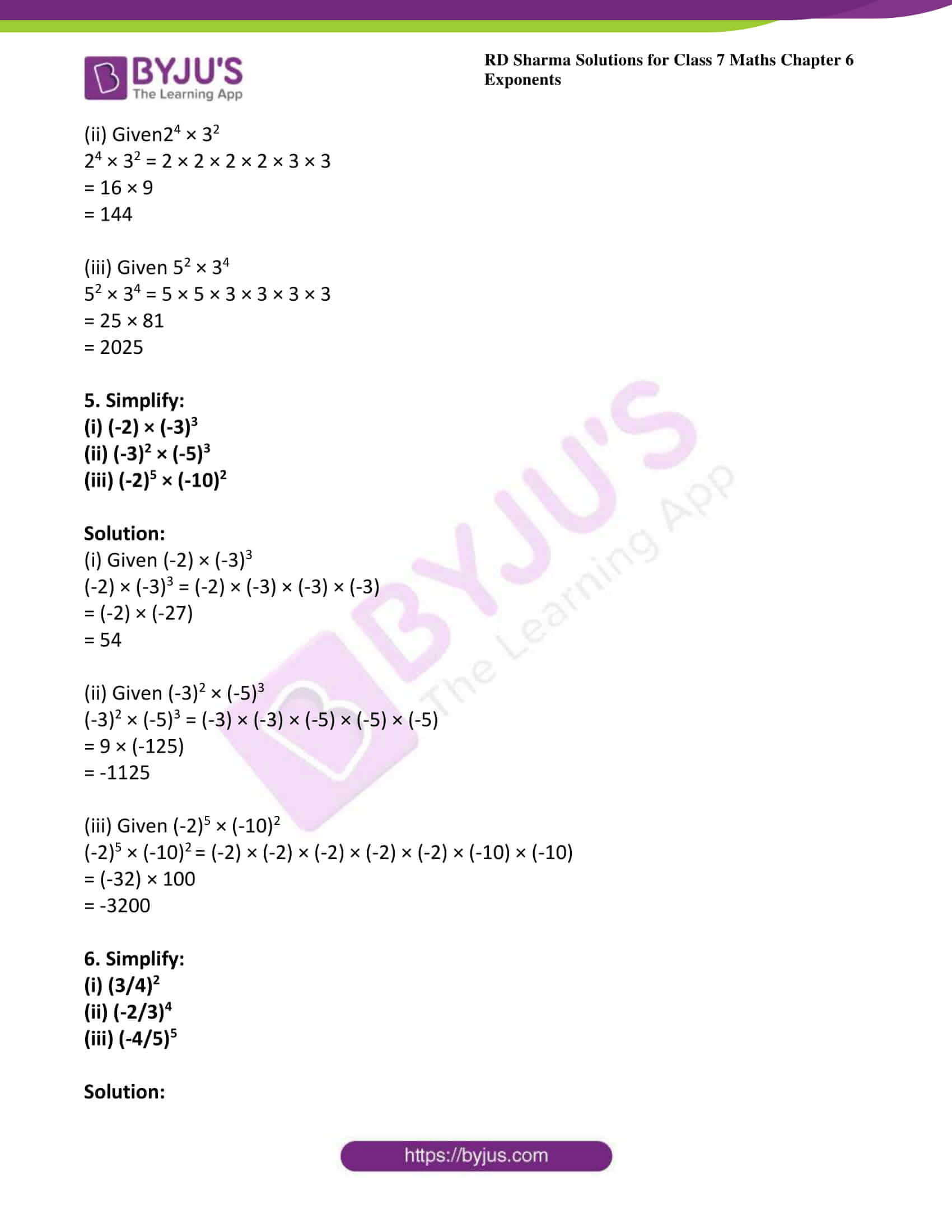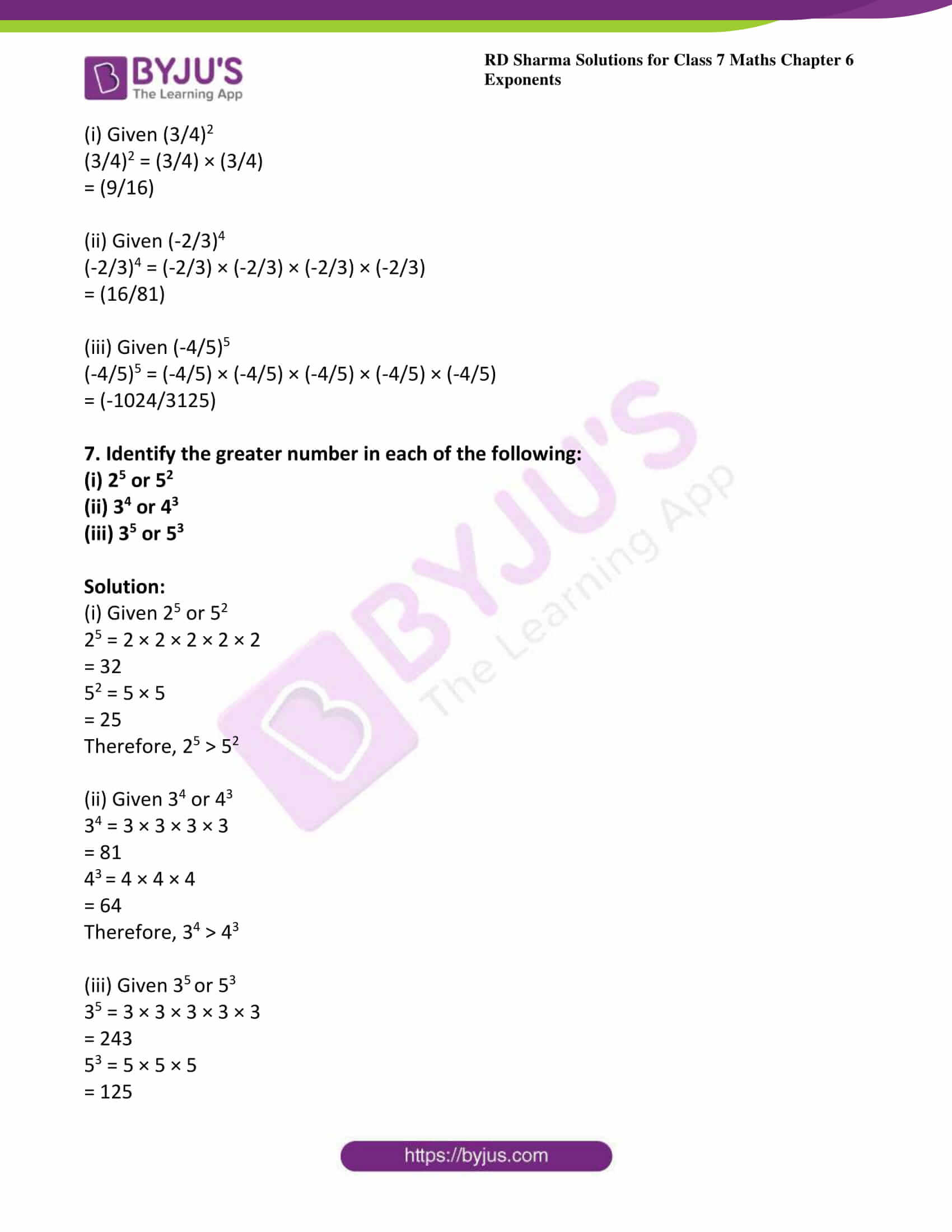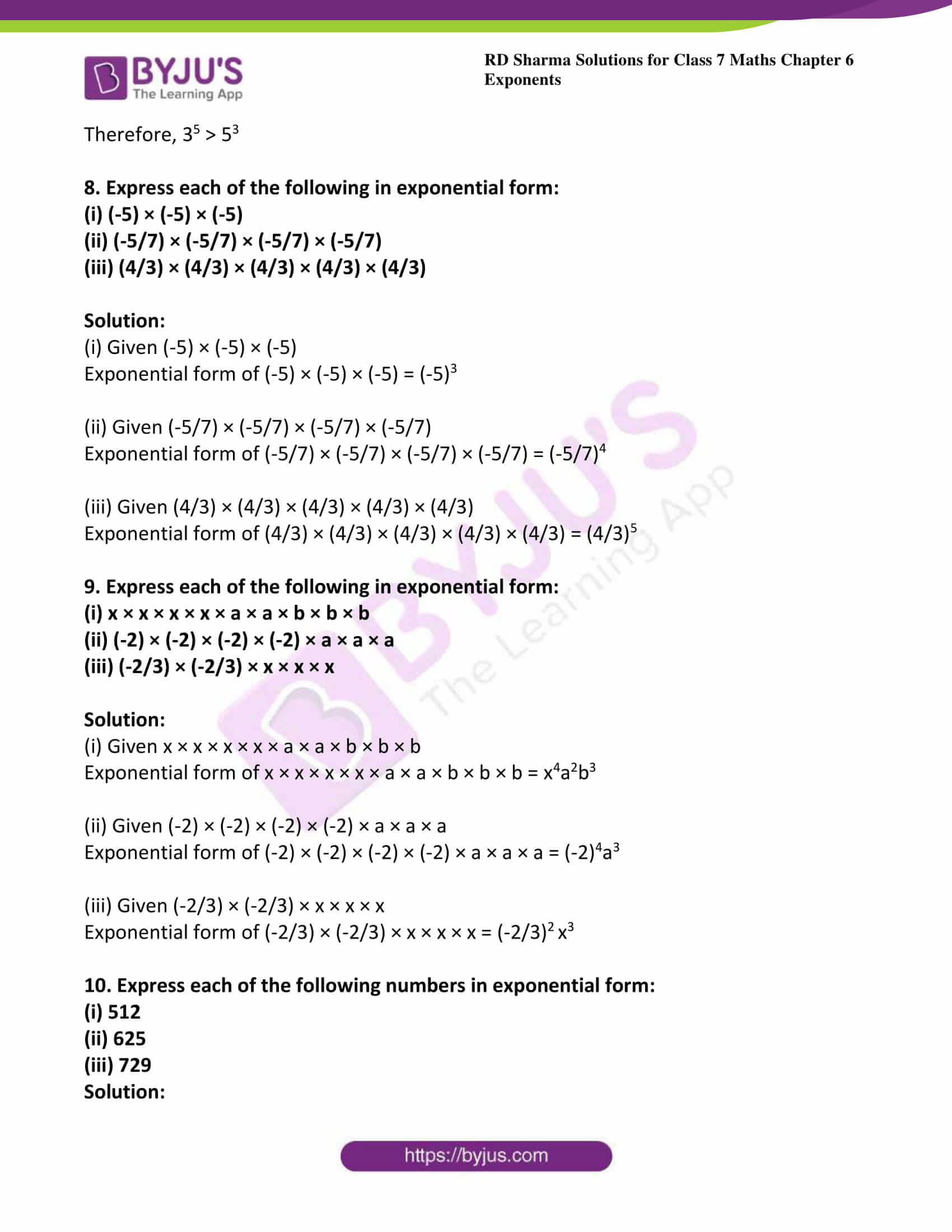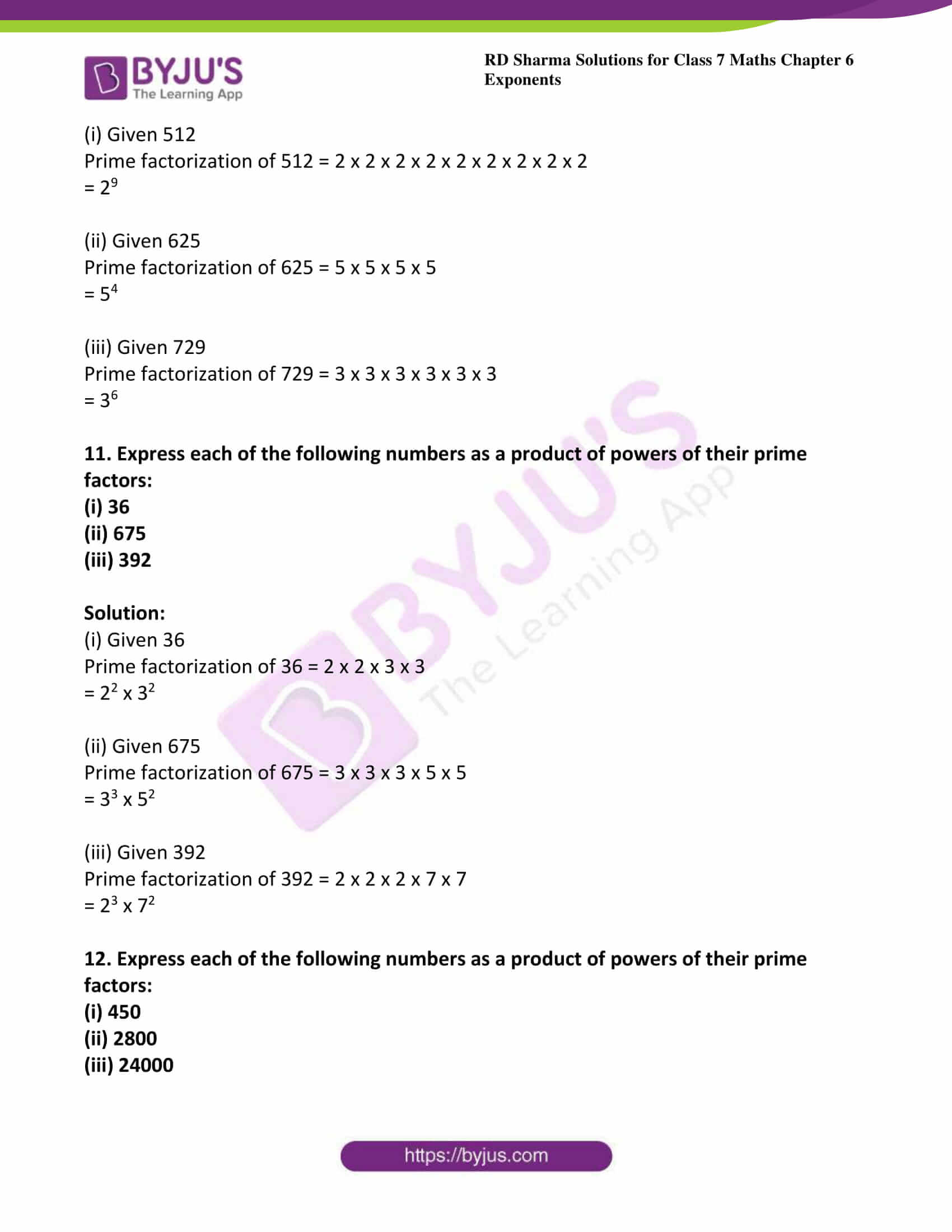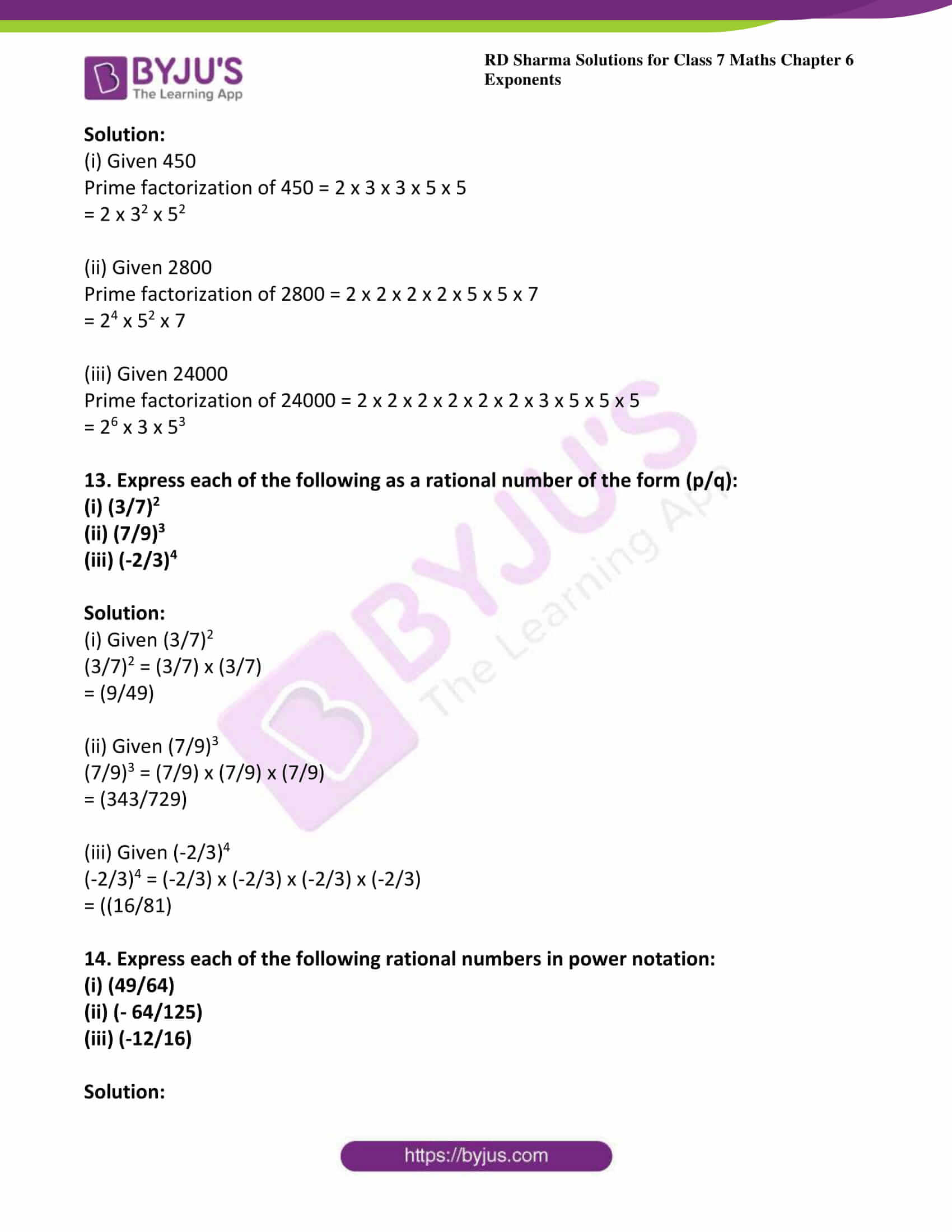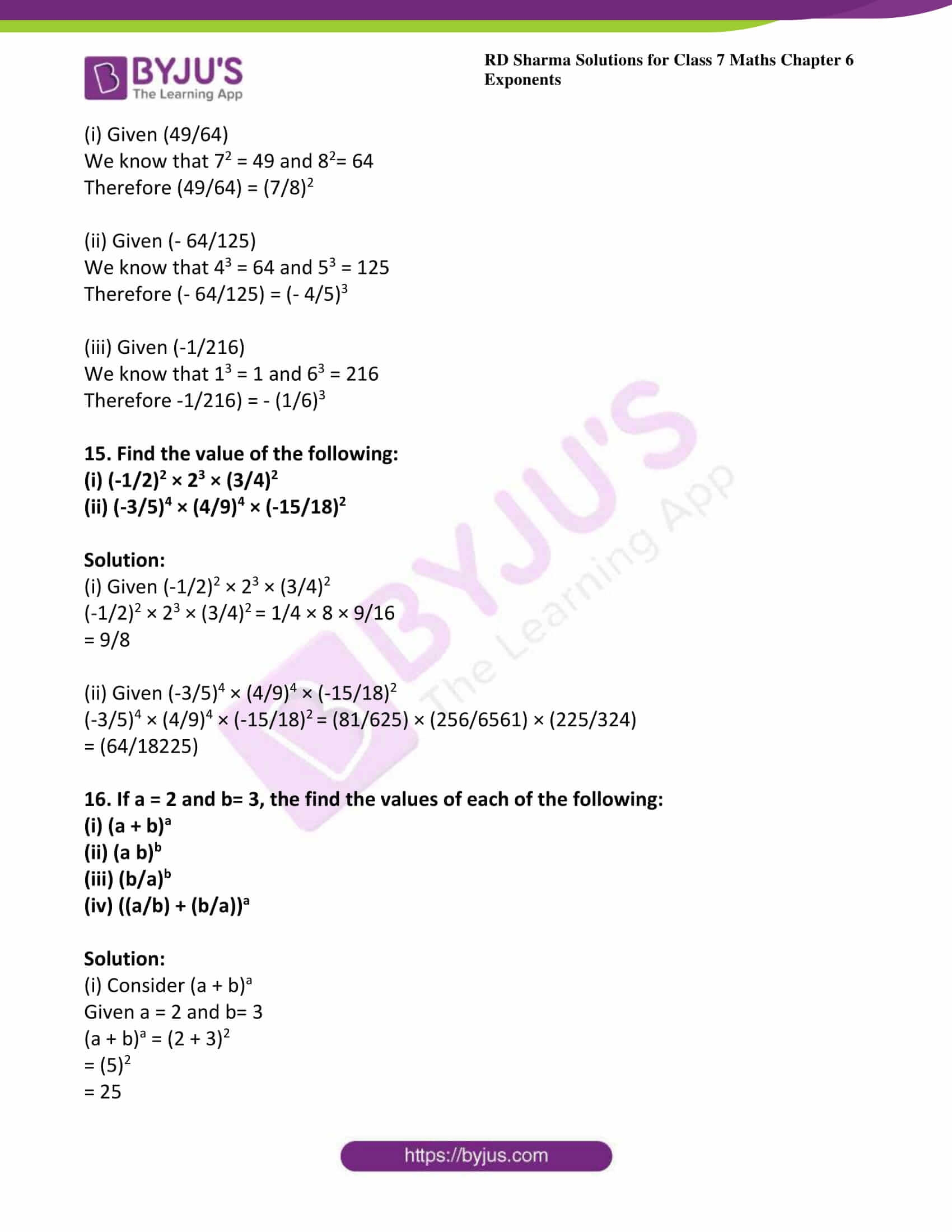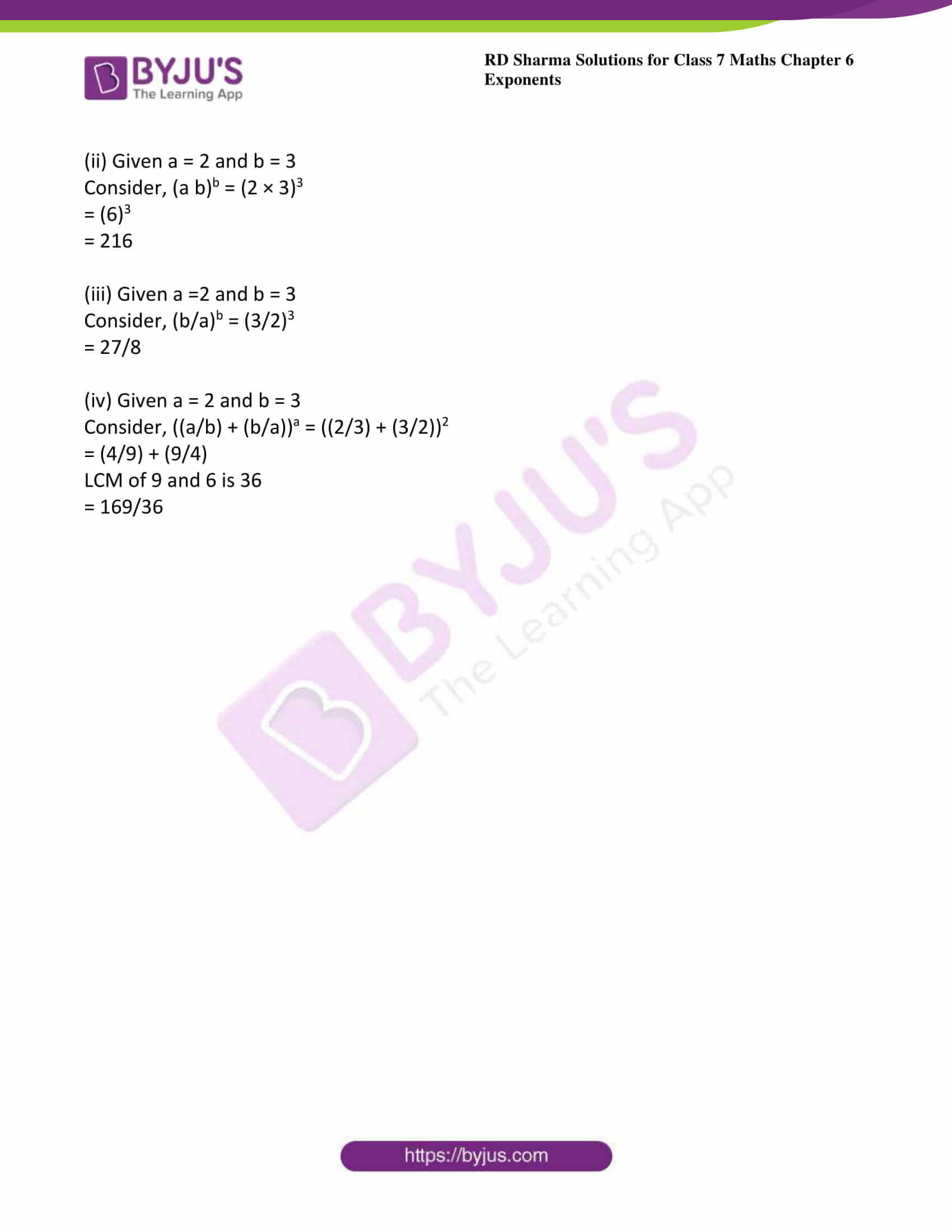### Access answers to Maths RD Sharma Solutions For Class 7 Chapter 6 – Exponents Exercise 6.1

1. Find the values of each of the following:

(i) 132

(ii) 73

(iii) 34

Solution:

(i) Given 132

132 = 13 × 13 =169

(ii) Given 73

73 = 7 × 7 × 7 = 343

(iii) Given 34

34 = 3 × 3 × 3 × 3

= 81

2. Find the value of each of the following:

(i) (-7)2

(ii) (-3)4

(iii)  (-5)5

Solution:

(i) Given (-7)2

We know that (-a) even number= positive number

(-a) odd number = negative number

We have, (-7)2 = (-7) × (-7)

= 49

(ii) Given (-3)4

We know that (-a) even number= positive number

(-a) odd number = negative number

We have, (-3)4 = (-3) × (-3) × (-3) × (-3)

= 81

(iii) Given (-5)5

We know that (-a) even number= positive number

(-a) odd number = negative number

We have, (-5)5 = (-5) × (-5) × (-5) × (-5) × (-5)

= -3125

3. Simplify:

(i) 3 × 102

(ii) 22 × 53

(iii) 33 × 52

Solution:

(i) Given 3 × 102

3 × 102 = 3 × 10 × 10

= 3 × 100

= 300

(ii) Given 22 × 53

22 × 53 = 2 × 2 × 5 × 5 × 5

= 4 × 125

= 500

(iii) Given 33 × 52

3× 52 = 3 × 3 × 3 × 5 × 5

= 27 × 25

= 675

4. Simply:

(i)  32 × 104

(ii)  24 × 32

(iii) 52 × 34

Solution:

(i)  Given 3× 104

3× 104 = 3 × 3 × 10 × 10 × 10 × 10

= 9 × 10000

= 90000

(ii) Given24 × 32

24 × 32 = 2 × 2 × 2 × 2 × 3 × 3

= 16 × 9

= 144

(iii) Given 52 × 34

52 × 34 = 5 × 5 × 3 × 3 × 3 × 3

= 25 × 81

= 2025

5. Simplify:

(i) (-2) × (-3)3

(ii) (-3)2 × (-5)3

(iii) (-2)5 × (-10)2

Solution:

(i) Given (-2) × (-3)3

(-2) × (-3)3 = (-2) × (-3) × (-3) × (-3)

= (-2) × (-27)

= 54

(ii) Given (-3)2 × (-5)3

(-3)2 × (-5)3 = (-3) × (-3) × (-5) × (-5) × (-5)

= 9 × (-125)

= -1125

(iii) Given (-2)5 × (-10)

(-2)5 × (-10)= (-2) × (-2) × (-2) × (-2) × (-2) × (-10) × (-10)

= (-32) × 100

= -3200

6. Simplify:

(i) (3/4)2

(ii) (-2/3)4

(iii) (-4/5)5

Solution:

(i) Given (3/4)2

(3/4)2 = (3/4) × (3/4)

= (9/16)

(ii) Given (-2/3)4

(-2/3)4 = (-2/3) × (-2/3) × (-2/3) × (-2/3)

= (16/81)

(iii) Given (-4/5)5

(-4/5)5 = (-4/5) × (-4/5) × (-4/5) × (-4/5) × (-4/5)

= (-1024/3125)

7. Identify the greater number in each of the following:

(i) 25 or 52

(ii) 34 or 43

(iii) 35 or 53

Solution:

(i) Given 25 or 52

25 = 2 × 2 × 2 × 2 × 2

= 32

52 = 5 × 5

= 25

Therefore, 25 > 52

(ii) Given 34 or 43

34 = 3 × 3 × 3 × 3

= 81

4= 4 × 4 × 4

= 64

Therefore, 34 > 43

(iii) Given 3or 53

35 = 3 × 3 × 3 × 3 × 3

= 243

53 = 5 × 5 × 5

= 125

Therefore, 35 > 53

8. Express each of the following in exponential form:

(i) (-5) × (-5) × (-5)

(ii) (-5/7) × (-5/7) × (-5/7) × (-5/7)

(iii) (4/3) × (4/3) × (4/3) × (4/3) × (4/3)

Solution:

(i) Given (-5) × (-5) × (-5)

Exponential form of (-5) × (-5) × (-5) = (-5)3

(ii) Given (-5/7) × (-5/7) × (-5/7) × (-5/7)

Exponential form of (-5/7) × (-5/7) × (-5/7) × (-5/7) = (-5/7)4

(iii) Given (4/3) × (4/3) × (4/3) × (4/3) × (4/3)

Exponential form of (4/3) × (4/3) × (4/3) × (4/3) × (4/3) = (4/3)5

9. Express each of the following in exponential form:

(i) x × x × x × x × a × a × b × b × b

(ii) (-2) × (-2) × (-2) × (-2) × a × a × a

(iii) (-2/3) × (-2/3) × x × x × x

Solution:

(i) Given x × x × x × x × a × a × b × b × b

Exponential form of x × x × x × x × a × a × b × b × b = x4a2b3

(ii) Given (-2) × (-2) × (-2) × (-2) × a × a × a

Exponential form of (-2) × (-2) × (-2) × (-2) × a × a × a = (-2)4a3

(iii) Given (-2/3) × (-2/3) × x × x × x

Exponential form of (-2/3) × (-2/3) × x × x × x = (-2/3)x3

10. Express each of the following numbers in exponential form:

(i) 512

(ii) 625

(iii) 729

Solution:

(i) Given 512

Prime factorization of 512 = 2 x 2 x 2 x 2 x 2 x 2 x 2 x 2 x 2

= 29

(ii) Given 625

Prime factorization of 625 = 5 x 5 x 5 x 5

= 54

(iii) Given 729

Prime factorization of 729 = 3 x 3 x 3 x 3 x 3 x 3

= 36

11. Express each of the following numbers as a product of powers of their prime factors:
(i) 36
(ii) 675
(iii) 392

Solution:

(i) Given 36

Prime factorization of 36 = 2 x 2 x 3 x 3

= 22 x 32

(ii) Given 675

Prime factorization of 675 = 3 x 3 x 3 x 5 x 5

= 33 x 52

(iii) Given 392

Prime factorization of 392 = 2 x 2 x 2 x 7 x 7

= 23 x 72

12. Express each of the following numbers as a product of powers of their prime factors:
(i) 450
(ii) 2800
(iii) 24000

Solution:

(i) Given 450

Prime factorization of 450 = 2 x 3 x 3 x 5 x 5

= 2 x 32 x 52

(ii) Given 2800

Prime factorization of 2800 = 2 x 2 x 2 x 2 x 5 x 5 x 7

= 24 x 52 x 7

(iii) Given 24000

Prime factorization of 24000 = 2 x 2 x 2 x 2 x 2 x 2 x 3 x 5 x 5 x 5

= 26 x 3 x 53

13. Express each of the following as a rational number of the form (p/q):

(i) (3/7)2

(ii) (7/9)3

(iii) (-2/3)4

Solution:

(i) Given (3/7)2

(3/7)2 = (3/7) x (3/7)

= (9/49)

(ii) Given (7/9)3

(7/9)3 = (7/9) x (7/9) x (7/9)

= (343/729)

(iii) Given (-2/3)4

(-2/3)4 = (-2/3) x (-2/3) x (-2/3) x (-2/3)

= ((16/81)

14. Express each of the following rational numbers in power notation:

(i) (49/64)

(ii) (- 64/125)

(iii) (-12/16)

Solution:

(i) Given (49/64)

We know that 72 = 49 and 82= 64

Therefore (49/64) = (7/8)2

(ii) Given (- 64/125)

We know that 43 = 64 and 53 = 125

Therefore (- 64/125) = (- 4/5)3

(iii) Given (-1/216)

We know that 13 = 1 and 63 = 216

Therefore -1/216) = – (1/6)3

15. Find the value of the following:

(i) (-1/2)2 × 23 × (3/4)2

(ii) (-3/5)4 × (4/9)4 × (-15/18)2

Solution:

(i) Given (-1/2)2 × 23 × (3/4)2

(-1/2)2 × 23 × (3/4)= 1/4 × 8 × 9/16

= 9/8

(ii) Given (-3/5)4 × (4/9)4 × (-15/18)

(-3/5)4 × (4/9)4 × (-15/18)= (81/625) × (256/6561) × (225/324)

= (64/18225)

16. If a = 2 and b= 3, the find the values of each of the following:

(i) (a + b)a

(ii) (a b)b

(iii) (b/a)b

(iv) ((a/b) + (b/a))a

Solution:

(i) Consider (a + b)a

Given a = 2 and b= 3

(a + b)a = (2 + 3)2

= (5)2

= 25

(ii) Given a = 2 and b = 3

Consider, (a b)b = (2 × 3)3

= (6)3

= 216

(iii) Given a =2 and b = 3

Consider, (b/a)b = (3/2)3

= 27/8

(iv) Given a = 2 and b = 3

Consider, ((a/b) + (b/a))a = ((2/3) + (3/2))2

= (4/9) + (9/4)

LCM of 9 and 6 is 36

= 169/36